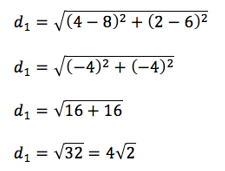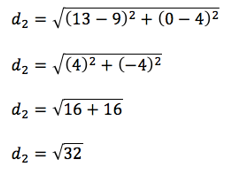PRIMARY

2 - 6

SECONDARY

7 - 10

SENIOR

11 - 12

Home

# Distance Formula

Theory & Practice Question.

## Lesson

#### [Formula]## Example

Show that the distance between the two points (8,6) and (4,2) is the same as the distance between the two points (9,4) and (13, 0).

## Solution

Recall the distance formulaLet x1 = 8,   x2 = 4,   y1 = 6,   y2 = 2Repeating the process:

Let x1 = 9,   x2 = 13,   y1 = 4,   y2 = 0Therefore:

d1 = d2

and so the distance between the two sets of points are equal

## Question

Determine the distance between the points (-5,-7) and (-3,1), simplifying your answer where necessary

## Explanation

The distance between 2 points (x1, y1) and (x2, y2) is found using the formulaWhen applied to the points (-5,-7) and (-3,1), we have